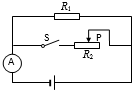$\text{A.}$ 电源电压为6V $\text{B.}$ 滑片P在M点时电流表的示数0.6A $\text{C.}$ 滑片P在移动过程中的最大阻值是20Ω $\text{D.}$ 滑片P在移动过程中的电路的最大功率是7.2W

C

#### 解析：

(2) 闭合开关 $S, R_1$ 与 $R_2$ 并联, 电流表测干路电流, 因并联电路中各支路独立工作、互不影响, 所以, 通过 $R_1$ 的电流保持不变,

$$\mathrm{I}_{\mathrm{M}}=\mathrm{I}_2 \mathrm{大}-\Delta \mathrm{I}=1 \mathrm{~A}-0.2 \mathrm{~A}=0.8 \mathrm{~A} \text {, }$$

(3) 当通过 $R_2$ 的电流最小时, 滑动变阻器接入电路中的电阻最大, 则通过 $R_2$ 的最小电路:
$$\mathrm{I}_{2 \text { 小 }}=\mathrm{I}_{\mathrm{M}}-\triangle \mathrm{I}=0.8 \mathrm{~A}-0.2 \mathrm{~A}=0.6 \mathrm{~A} \text {, }$$

$$\mathrm{R}_2 \text { 大 }=\frac{\mathrm{U}}{\mathrm{I}_2 \text { 小 }}=\frac{12 \mathrm{~V}}{0.6 \mathrm{~A}}=20 \Omega \text {, 故C正确; }$$
(4) 当通过 $R_2$ 的电流最大时, 电路的功率最大,

$\mathrm{P} 大=U I_大=12 \mathrm{~V} \times 1.4 \mathrm{~A}=16.8 \mathrm{~W}$, 故D错误。

①点击 收藏 此题， 扫码注册关注公众号接收信息推送（一月四份试卷,中1+高2+研1）
② 程序开发、服务器资源都需要大量的钱，如果你感觉本站好或者受到到帮助，欢迎赞助本站,赞助方式：微信/支付宝转账到 18155261033

 ①此题难易度如何 ②此题推荐度如何 确定